Translations
Reflections
Rotations
Congruence and Transformations
Dilations
Similarity and Transformations
100

Translate △XYZ with vertices X(2, 3), Y(−3, 2), and Z(−4, −3) by (x, y) → (x, y + 2)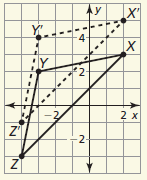X'(2, 5), Y'(−3, 4), and Z'(−4, −1)

100

△ABC has vertices A(1, 3), B(4, 1), and C(1, 1).

Graph △ABC and its image after a reflection over the line y = x.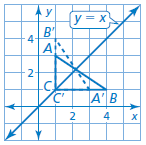A'(3,1), B'(1,4), and C'(1, 1)

100

Graph the polygon with the given vertices and its image after a rotation of the given number of degrees about the origin.

A(−3, −1), B(2, 2), C(3, −3); 90°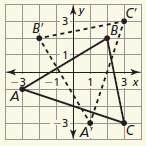100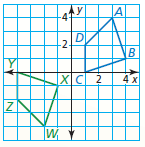A congruence transformation that maps quadrilateral ABCD to quadrilateral WXYZ is a reflection in the x-axis followed by a translation of 5 units left.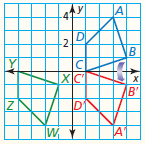100

Find the scale factor of the dilation. Then tell whether it is a reduction or an enlargement.k = 5/12, reduction

100

Line AB has endpoints A(−8, 6) and B(6, 0). Find the endpoints of its image after the similarity transformation.

Translation: (x, y) → (x, y − 6)

Dilation: (x, y) → (1/2x, 1/2y)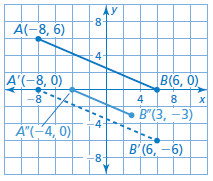200

Translate △XYZ with vertices X(2, 3), Y(−3, 2), and Z(−4, −3) by (x, y) → (x − 3, y)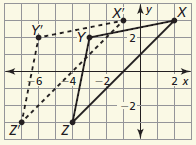X'(-1, 3), Y'(−6, 2), and Z'(−7, −3)

200

How many lines of symmetry does the figure have?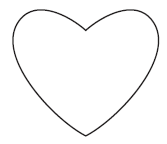1

200

Graph the polygon with the given vertices and its image after a rotation of the given number of degrees about the origin.

W(−2, −1), X(−1, 3), Y(3, 3), Z(3, −3); 180°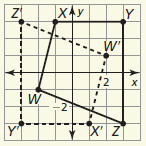200

Describe a congruence transformation that maps △DEF to △JKL.

D(2, −1), E(4, 1), F(1, 2)

J(−2, −4), K(−4, −2), L(−1, −1)

reflection in the y-axis followed by a translation

3 units down

200

Graph △DEF with vertices D(2, 6), E(2, 2), and F(4, 2) and its image after a dilation with scale factor -1/2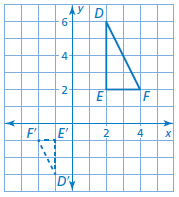200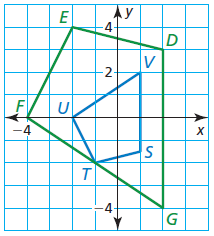A reflection in the x-axis followed by a dilation with a scale factor of 2

300

Translate △XYZ with vertices X(2, 3), Y(−3, 2), and Z(−4, −3) by (x, y) → (x + 3, y − 1)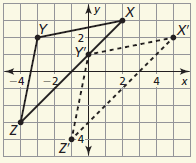X'(5, 2), Y'(0, 1), and Z'(−1, −4)

300

Graph the polygon and its image after a reflection over the given line, x=4.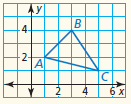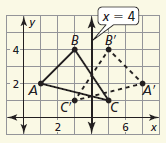300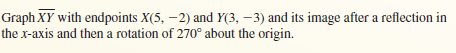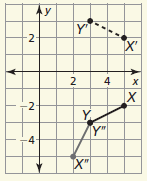300

Describe a congruence transformation that maps △DEF to △JKL.

D(−3, −4), E(−5, −1), F(−1, 1)

J(1, 4), K(−1, 1), L(3, −1)

180° rotation about the origin followed by a reflection in the line x = 2

300

Graph the triangle and its image after a dilation with scale factor k.

P(2, 2), Q(4, 4), R(8, 2); k = 1/2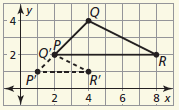300

Describe a similarity transformation that maps △ABC to △RST.

A(1, 0), B(−2, −1), C(-1, -2)

R(-3, 0), S(6, -3), T(3, −6)

reflection in the line x = −1 followed by a dilation with center (−3, 0) and k = 3

400

Translate △XYZ with vertices X(2, 3), Y(−3, 2), and Z(−4, −3) by (x, y) → (x + 4, y + 1)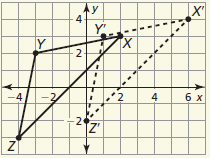X'(6, 4), Y'(1, 3), and Z'(0, −2)

400

Graph the polygon and its image after a reflection in the given line, y=3.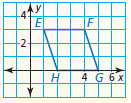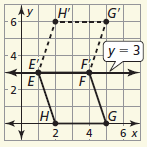400

Determine whether the figure has rotational symmetry. If so, describe any rotations that map the figure onto itself.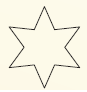yes; Rotations of 60°, 120°, and 180° about the center map the figure onto itself.

400

Which transformation is the same as reflecting an object in two parallel lines?

translation

400

Graph the triangle and its image after a dilation with scale factor k.

X(−3, 2), Y(2, 3), Z(1, −1); k = −3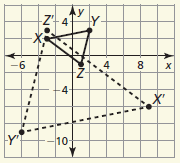400

Describe a similarity transformation that maps △ABC to △RST.

A(6, 4), B(−2, 0), C(−4, 2)

R(2, 3), S(0, −1), T(1, −2)

dilation with center at the origin and k = 1/2, followed by a reflection in the line y = x

500

What are the vertices of △P"Q"R" with vertices P(0, −4), Q(1, 3), and R(2, −5) after the composition

Translation: (x, y) → (x + 1, y + 2)

Translation: (x, y) → (x − 4, y + 1)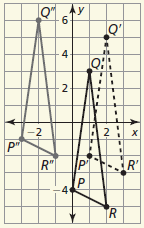P'(1, −2), Q'(2, 5), and R'(3, −3)

P''(-3, −1), Q''(-2, 6), and R''(-1, −2)

500

How many lines of symmetry does the figure have?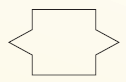2

500

Determine whether the figure has rotational symmetry. If so, describe any rotations that map the figure onto itself.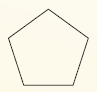yes; Rotations of 72° and 144° about the center map the figure onto itself.

500

Which transformation is the same as reflecting an object in two intersecting lines?

rotation

500

You are using a magnifying glass that shows the image of an object that is eight times the object’s actual size. The image length is 15.2 centimeters. Find the actual length of the object.

1.9 cm

500

Describe a similarity transformation that maps △ABC to △RST.

A(3, −2), B(0, 4), C(−1, −3)

R(−4, −6), S(8, 0), T(−6, 2)

270° rotation about the origin followed by a dilation with center at the origin and k = 2

Click to zoom